# MCAT Physical : Solution Chemistry

## Example Questions

← Previous 1 3 4 5 6 7 8

### Example Question #1 : Ions In Solution

A U-shaped tube is filled with water and then split into two sections by a membrane at the lowest part of the tube. The membrane is permeable to water, but is impermeable to ions. 50g of salt are added to the left side of the tube and allowed to enter solution.

Which of the following would not result following the addition of salt?

Possible Answers:

The concentrations of both sides will become equal as water flows through the membrane.

The forces of entropy and pressure will be equal when the solution is at equilibrium.

The osmotic pressure of the left side will be greater than the right side.

The water will be higher on the side that has salt.

Correct answer:

The concentrations of both sides will become equal as water flows through the membrane.

Explanation:

Osmotic pressure is defined as the tendency for water to diffuse into a solution via osmosis. Since the membrane is not permeable to ions, the salt ions are unable to cross the membrane and make the concentrations equal on both sides of the tube. Instead, water will flow to the side that has salt until the pressure in the tube with salt equals the forces of entropy. This will result in a higher level of water on the side of the tube with salt in it.

### Example Question #1 : Solubility And Ions

The Haber-Bosch process, or simply the Haber process, is a common industrial reaction that generates ammonia from nitrogen and hydrogen gas. A worker in a company generates ammonia from the Haber process. He then dissociates the gaseous ammonia in water to produce an aqueous solution. Since ammonia is a base, it will accept a proton from water, generating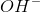and ammonium ion products. The two reactions involved are: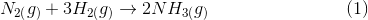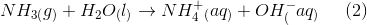The ammonium ion generated from the dissociation of ammonia is __________.

Possible Answers:

a cation because it loses an electron

an anion because it gains an electron

a cation because it gains an electron

an anion because it loses an electron

Correct answer:

a cation because it loses an electron

Explanation:

Remember that there are two types of ions: cations and anions. Cations are produced when a atom of a molecule loses an electron. A neutral atom will contain no charge; however, when the atom loses an electron the atom becomes positively charged because it will contain more protons than electrons. In reaction 2, the nitrogen in ammonia loses an electron (because it shares the electron with the new hydrogen atom), which produces a positively charged ammonium ion. The ammonium ion is a cation because of this positive charge.

Anions are formed when an atom gains an electron, which makes the overall charge of an atom negative. The hydroxide ions from reaction 2 are anions.

### Example Question #1 : Ions In Solution

Which of the following solutions is NOT a good electrolyte?

Possible Answers:

NaCl

CH3COOH

NaOH

CO2

Correct answer:

CO2

Explanation:

Electrolyte solutions are formed when a compound creates ions once in solution. Carbon dioxide will not create ions in solution, so it is not a good electrolyte.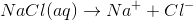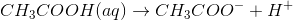(This is the acid dissociation for acetic acid.)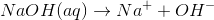### Example Question #1 : Solution Chemistry

What is the Vant Hoff factor of the molecule Li3PO4?

Possible Answers:Correct answer:Explanation:

The Vant Hoff factor indicates how many particles a solid produces when dissolved in solution. When Li3PO4dissolves in solution, there are three Li+ molecules and one molecule of PO4-3. The Vant Hoff factor is equal to the sum of molecules: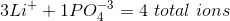.

### Example Question #2 : Ions In Solution

Hydrochloric acid and sodium sulfite react in aqueous solution via the process: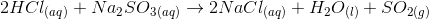Which of the following correctly expresses the net ionic equation for this reaction?

Possible Answers:Correct answer:Explanation:

Start by writing the total ionic equation, using the following steps.

1. Break all soluble strong electrolytes, denoted by "(aq)," into their ions, indicating the number and charge of each ion.

2. Bring along unchanged any compounds denoted as "(s)," "(l)," or "(g)."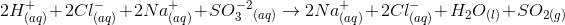Remove any ions appearing on both sides of the reaction (any "spectator" ions) to get the net ionic equation.

Spectator ions: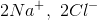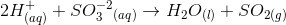### Example Question #1 : Solution Chemistry

All of the following are true regarding a solution except __________.

Possible Answers:

An under-saturated solution is a solution that contains less solute than the solvent is capable of dissolving

A solution can contain any of the three phases of matter

A solution is composed of a solute in one phase dissolved in a solvent in another phase

A saturated solution is in a state of equilibrium

Solvation is the process by which a solute dissolves into a solvent and becomes a solution

Correct answer:

A solution is composed of a solute in one phase dissolved in a solvent in another phase

Explanation:

Solutions can occur in all three phases of matter. They can also occur between two compounds in a single phase. For example, brass is a solution of two metals: zinc and copper.

### Example Question #1 : Solution Chemistry

Electrolytes play a big role in maintaining blood pressure. Loss of electrolytes often leads to a drop in blood pressure. What can a doctor prescribe to a patient who has very low blood pressure?

Possible Answers:

Increase the consumption of lithium chloride

Increase the consumption of sodium chloride

Decrease the consumption of salt

Spend an hour a day in a hot sauna

Correct answer:

Increase the consumption of sodium chloride

Explanation:

The question states that the patient has a low blood pressure, suggesting that the patient has a low concentration of electrolytes in his blood. To counter this, the doctor must prescribe a solution that will increase the concentration of electrolytes in blood. The best solution would be to increase the consumption of salt, or sodium chloride. Sodium chloride is a strong electrolyte and will dissociate into sodium ions and chlorine ions. This will increase the blood pressure of the patient and will remedy the problem.

Spending an hour a day in a hot sauna will cause excessive sweating. Remember that salts are excreted through sweat; therefore, the electrolyte concentration, and the patient's blood pressure, will decrease further if the patient spends time in a sauna. Although lithium chloride is a strong electrolyte (like sodium chloride), the doctor will not prescribe lithium chloride because it is a toxic substance.

### Example Question #1 : Solubility And Ions

Adding ammonia to a solution containing a copper hydroxide precipitate has the effect of dissolving the precipitate because __________.

Possible Answers:

hydroxide ions bond ionically with ammonia

the addition of ammonia allows more hydroxide and hydrogen ions to form water molecules, increasing the solubility of copper hydroxide

covalent bonds form between copper ions and ammonia molecules

an increase in hydronium ions shifts the reaction of copper and hydroxide to the left

Correct answer:

covalent bonds form between copper ions and ammonia molecules

Explanation:

The copper ion is able to form four covalent bonds. This can be explained with electron configurations.

The copper atom configuration: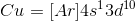The copper ion configuration: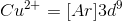The copper ion can accept electrons that fill empty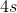and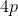levels. In this example, the chemical equation is as follows: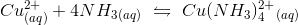### Example Question #1 : Solution Chemistry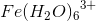and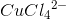are alike in that __________.

Possible Answers:

both compounds are able to form coordinate covalent bonds with a metal ion

both involve coordinate covalent bonds that utilize empty electron orbitals in the metal ion

both involve hybridization of the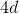energy level

both involve ionic bonds that form due to polarity

Correct answer:

both involve coordinate covalent bonds that utilize empty electron orbitals in the metal ion

Explanation:

Complex ions typically form when coordinate covalent bonds are formed between ligands, such as water or chloride, and metal ions that have lost electrons and have available orbitals. While one answer choice, "Both compounds are able to form coordinate covalent bonds with a metal ion," may seem correct, this happens within the compound. The compounds themselves are ions that will form ionic compounds.

### Example Question #1 : Solution Chemistry

Which is an inaccurate depiction of the central iron atom in a hemoglobin molecule?

Possible Answers:

It has a coordination number of 6.

It is covalently bonded to five water molecules that can easily be replaced with oxygen molecules.

It is able to form coordinate covalent bonds with water, oxygen, and carbon monoxide.

It is covalently bonded to five nitrogen atoms, one of which is a component of the globin protein.

Correct answer:

It is covalently bonded to five water molecules that can easily be replaced with oxygen molecules.

Explanation:

The central iron in hemoglobin is the middle of a complex compound and is involved in six covalent bonds (coordination number of 6). It is bonded to five nitrogen atoms, one of which is part of an amino acid in the globin protein. This leaves one remaining bond, which can be with water, oxygen, or carbon monoxide (which forms a very stable bond, making it toxic).

← Previous 1 3 4 5 6 7 8

### All MCAT Physical Resources